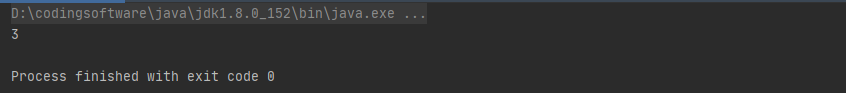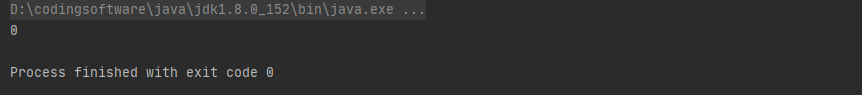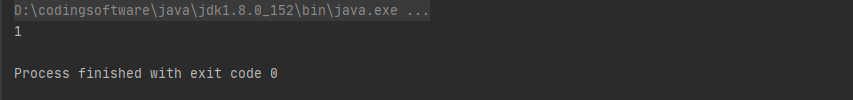# 如何在Java中避免建立不必要的物件（備戰2022春招或暑期實習，每天進步一點點，打卡100天，Day1）

• 備戰2022春招或暑期實習，祝大家每天進步億點點！Day1
• 本篇總結的是 如何在Java中避免建立不必要的物件，後續會每日更新~
• 關於《Redis入門到精通》、《併發程式設計》等知識點可以參考我的往期部落格：xxx
• 相信自己，越活越堅強，活著就該逢山開路，遇水架橋！生活，你給我壓力，我還你奇蹟！## 簡介## 1、String和Boolean

```// 每次都會建立一個新的String物件，且不會加入常量池 String name2 = new String("李子捌");```

```// 正確寫法 String name1 = "李子捌";```

``` // StringBuffer中的append()方法 public synchronized StringBuffer append(String str) { toStringCache = null; super.append(str); return this; }

// StringBuilder中的append()方法 public StringBuilder append(String str) { super.append(str); return this; } ```

Boolean是常用的型別，在開發中也應該使用Boolean.valueof()而不是new Boolean()，從Boolean的原始碼可以看出，Boolean類定義了兩個final static的屬性，而Boolean.valueof()直接返回的是定義的這兩個屬性，而new Boolean()卻會建立新的物件。

``` public static final Boolean TRUE = new Boolean(true);

public static final Boolean FALSE = new Boolean(false); ```

## 2、自動拆箱和裝箱

Java提供了基本資料型別的自動拆箱和裝箱功能，那是不是意味著我們可以在程式碼中隨意的使用這兩個型別呢？其實理論上在程式碼層面是沒得問題，不過在具體的效能方面還是有優化的空間啦！！！

```long start = System.currentTimeMillis(); Integer sum = 0; for (int i = 0; i < 100000; i++) { sum += i; } System.out.println(System.currentTimeMillis() - start);``````long start = System.currentTimeMillis(); // 修改Integer 為 int int sum = 0; for (int i = 0; i < 100000; i++) { sum += i; } System.out.println(System.currentTimeMillis() - start);```## 3、正則表示式

``` public static void main(String[] args) {

``````String email = "[email protected]";
String regex = "^([a-z0-9A-Z]+[-|\.]?)+[a-z0-9A-Z]@([a-z0-9A-Z]+(-[a-z0-9A-Z]+)?\.)+[a-zA-Z]{2,}\$";

long start = System.currentTimeMillis();
for (int i = 0; i < 10000; i++) {
email.matches(regex);
}

System.out.println(System.currentTimeMillis() - start);
``````

} `````` public static void main(String[] args) {

``````String email = "[email protected]";
String regex = "^([a-z0-9A-Z]+[-|\.]?)+[a-z0-9A-Z]@([a-z0-9A-Z]+(-[a-z0-9A-Z]+)?\.)+[a-zA-Z]{2,}\$";
Pattern pattern = Pattern.compile(regex);

long start = System.currentTimeMillis();
for (int i = 0; i < 10000; i++) {
//email.matches(regex);
pattern.matcher(email);
}

System.out.println(System.currentTimeMillis() - start);
``````

} ```「歡迎在評論區討論，掘金官方將在掘力星計劃活動結束後，在評論區抽送100份掘金周邊，抽獎詳情見活動文章」